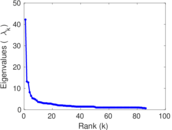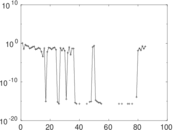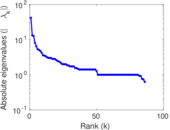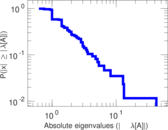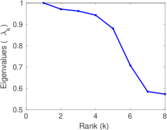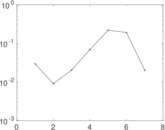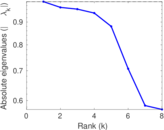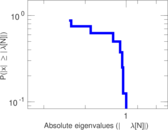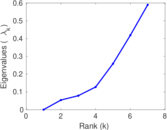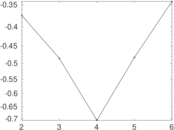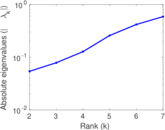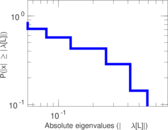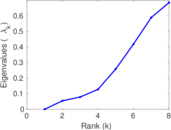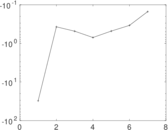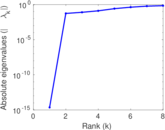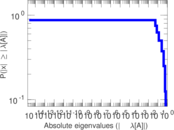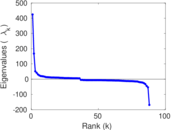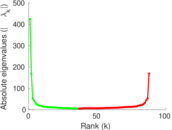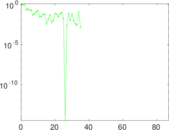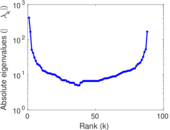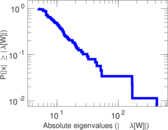# Wikibooks edits (su)

This is the bipartite edit network of the Sundanese Wikibooks. It contains users and pages from the Sundanese Wikibooks, connected by edit events. Each edge represents an edit. The dataset includes the timestamp of each edit.

 Code `bsu` Internal name `edit-suwikibooks` Name Wikibooks edits (su) Data source http://dumps.wikimedia.org/ AvailabilityDataset is available for download Consistency checkDataset passed all tests Category Authorship network Dataset timestamp 2017-10-20 Node meaning User, article Edge meaning Edit Network formatBipartite, undirected Edge typeUnweighted, multiple edges Temporal dataEdges are annotated with timestamps

## Statistics

 Size n = 433 Left size n1 = 88 Right size n2 = 345 Volume m = 525 Unique edge count m̿ = 392 Wedge count s = 8,822 Claw count z = 335,723 Cross count x = 10,340,072 Square count q = 95 4-Tour count T4 = 36,916 Maximum degree dmax = 138 Maximum left degree d1max = 138 Maximum right degree d2max = 42 Average degree d = 2.424 94 Average left degree d1 = 5.965 91 Average right degree d2 = 1.521 74 Fill p = 0.012 911 7 Average edge multiplicity m̃ = 1.339 29 Size of LCC N = 162 Diameter δ = 7 50-Percentile effective diameter δ0.5 = 1.736 92 90-Percentile effective diameter δ0.9 = 3.726 67 Median distance δM = 2 Mean distance δm = 2.638 99 Gini coefficient G = 0.583 743 Balanced inequality ratio P = 0.269 524 Left balanced inequality ratio P1 = 0.220 952 Right balanced inequality ratio P2 = 0.390 476 Relative edge distribution entropy Her = 0.866 444 Power law exponent γ = 5.467 05 Tail power law exponent γt = 2.721 00 Tail power law exponent with p γ3 = 2.721 00 p-value p = 0.231 000 Left tail power law exponent with p γ3,1 = 1.841 00 Left p-value p1 = 0.045 000 0 Right tail power law exponent with p γ3,2 = 3.611 00 Right p-value p2 = 0.043 000 0 Degree assortativity ρ = −0.255 864 Degree assortativity p-value pρ = 2.815 32 × 10−7 Spectral norm α = 42.201 9 Algebraic connectivity a = 0.053 987 8 Spectral separation |λ1[A] / λ2[A]| = 3.199 72 Controllability C = 259 Relative controllability Cr = 0.600 928

## Plots

### Fruchterman–Reingold graph drawing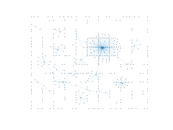### Degree distribution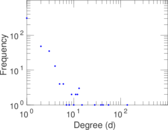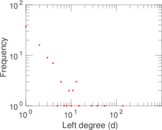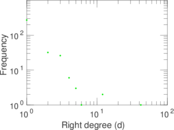### Cumulative degree distribution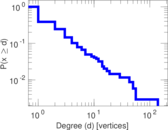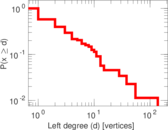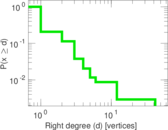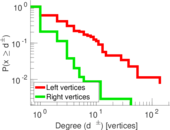### Lorenz curve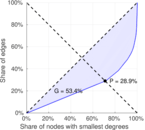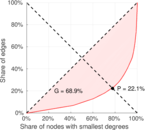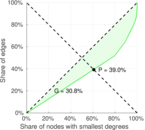### Spectral distribution of the adjacency matrix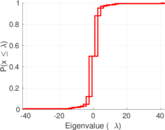### Spectral distribution of the normalized adjacency matrix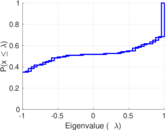### Spectral distribution of the Laplacian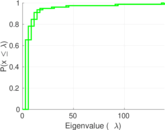### Spectral graph drawing based on the adjacency matrix### Spectral graph drawing based on the Laplacian### Spectral graph drawing based on the normalized adjacency matrix### Degree assortativity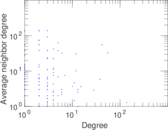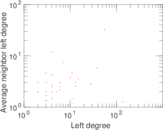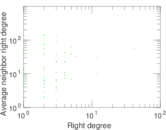### Zipf plot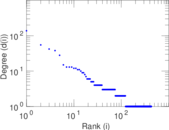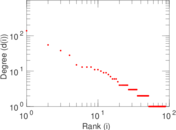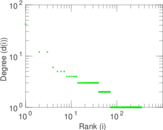### Hop distribution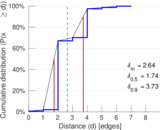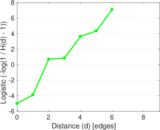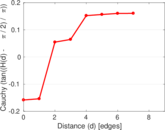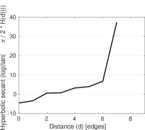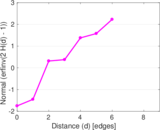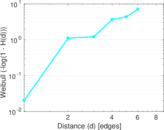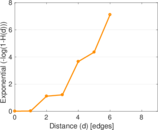### Double Laplacian graph drawing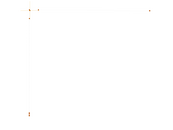### Delaunay graph drawing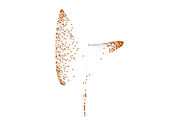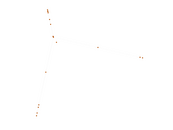### Edge weight/multiplicity distribution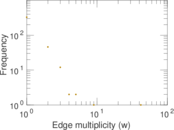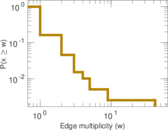### Temporal distribution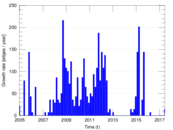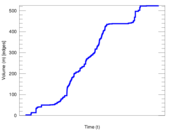### Temporal hop distribution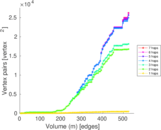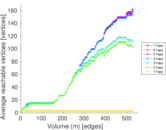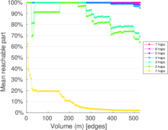### Diameter/density evolution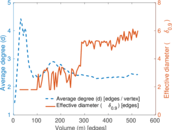### Matrix decompositions plots